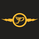4432 views
Returns a dashboard showing the direction taken by 4 overlay indicators, SMA ( simple moving average ), TMA (triangular moving average), WMA ( weighted moving average ), and REG ( linear regression ), all using different length periods.

The user can select the minimum and maximum length of these indicators and introduce an increment.

1. Settings

• Maximum Length: The end value of sequences of the indicator periods to analyze
• Minimum Length: The starting value of sequences of the indicator periods to analyze
• Step: Determines the spacing between each indicator periods values
• Src: Data source for each of the 4 indicators

1.1 Style settings

• Normalized Change Mode: Allows the user to access a different interpretation of the indicator by showing the normalized first differences of each indicator in the dashboard instead of their sign
• Dashboard Location: Location of the dashboard on the chart
• Dashboard Size: Size of the dashboard on the chart
• Text/Frame Color: Determines the color of the frame grid as well as the text color
• Bullish Cell Color: Determines the color of cell associated with a rising indicator direction
• Bearish Cell Color: Determines the color of cell associated with a decreasing indicator direction
• Cell Transparency: Transparency of each cell

2. Usage

Each of the indicators included in the dashboard aim to give an estimate of the underlying trend in the price. Knowing which direction they are taking can help us have a broader view regarding the direction of shorter/longer-term trends. We will later see that this is not the only kind of information that we can get from this indicator.

Rising indicators are represented by blue cells (or the color selected in the Bullish Cell Color setting) while decreasing indicators are represented by red cells (or the color selected in the Bearish Cell Color setting).

The percentage of bullish cells is given in the top-left cell of the dashboard.

2.1 Normalized change mode

Enabling the Normalized Change mode will display the normalized changes returned by the indicators over different length periods. This metric is within a range (0,1), with 1 indicating the highest change over the selected length periods, while 0 indicates the lowest one.

When enabling this mode the color of the cells makes use of a gradient with a color palette ranging from the color selected in the Bearish Cell setting to the color selected in the Bullish Cell setting.

2.1 Other Usage

The direction taken by certain indicators can give more information than one would think. Indeed, the sign of the change of one indicator can often be given by different indicators.

A positive change in a simple moving average indicates that the price is greater than the price p bars ago, where p is the period of the simple moving average .

A positive change in a triangular moving average indicates that a simple moving average of period p is above a simple moving average of period p × 2, where p is the period of the triangular moving average (note that we assume here that the TMA is given by cascading two SMAs of period p).

A positive change in a weighted moving average indicates that the price is above a simple moving average of period p+1, where p is the period of the WMA .

Finally, a positive change in a linear regression indicates that a weighted moving average is above a simple moving average of period p, where p is the period of the linear regression .
Release Notes: Thumbnail update for the aesthetics
Open-source script

In true TradingView spirit, the author of this script has published it open-source, so traders can understand and verify it. Cheers to the author! You may use it for free, but reuse of this code in a publication is governed by House Rules. You can favorite it to use it on a chart.

Want to use this script on a chart?

Full guides & documentation: https://docs.luxalgo.com